# Explain Image Classification by SHAP Deep Explainer

## Goal¶

This post aims to introduce how to explain Image Classification (trained by PyTorch) via SHAP Deep Explainer.

Shap is the module to make the black box model interpretable. For example, image classification tasks can be explained by the scores on each pixel on a predicted image, which indicates how much it contributes to the probability positively or negatively.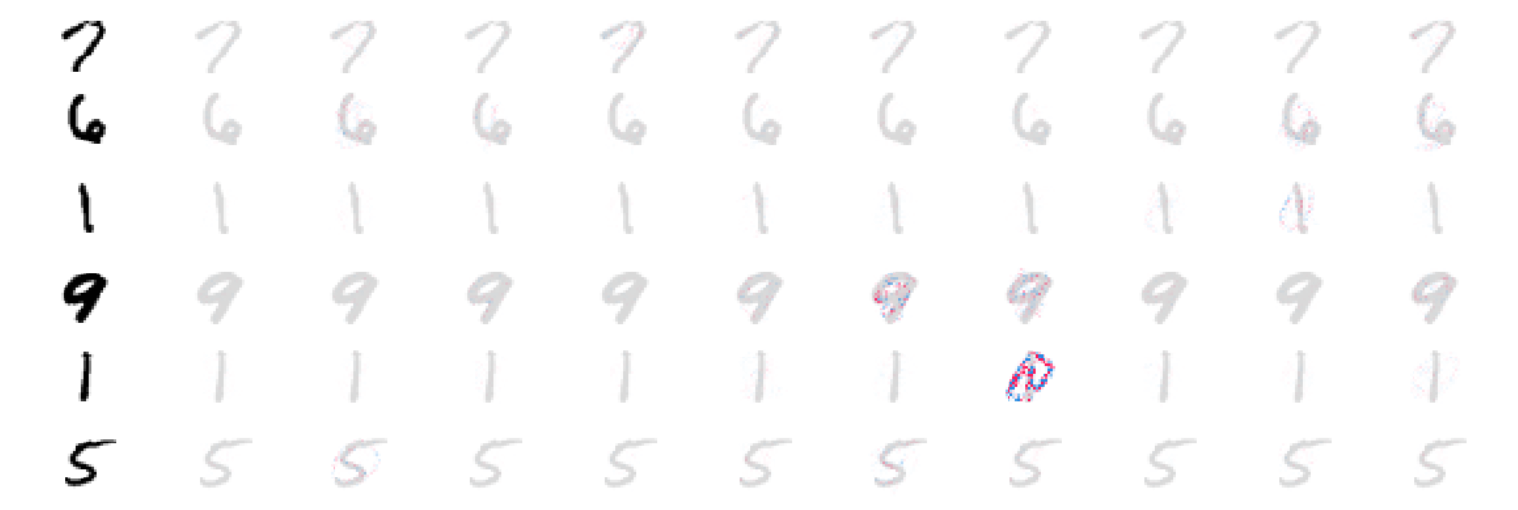Reference

# Loss Functions in Deep Learning with PyTorch

## Goal¶

This post aims to compare loss functions in deep learning with PyTorch.

The following loss functions are covered in this post:

• Mean Absolute Error (L1 Loss)
• Mean Square Error (L2 Loss)
• Binary Cross Entropy (BCE)
• Kullback-Leibler divergence (KL divergence)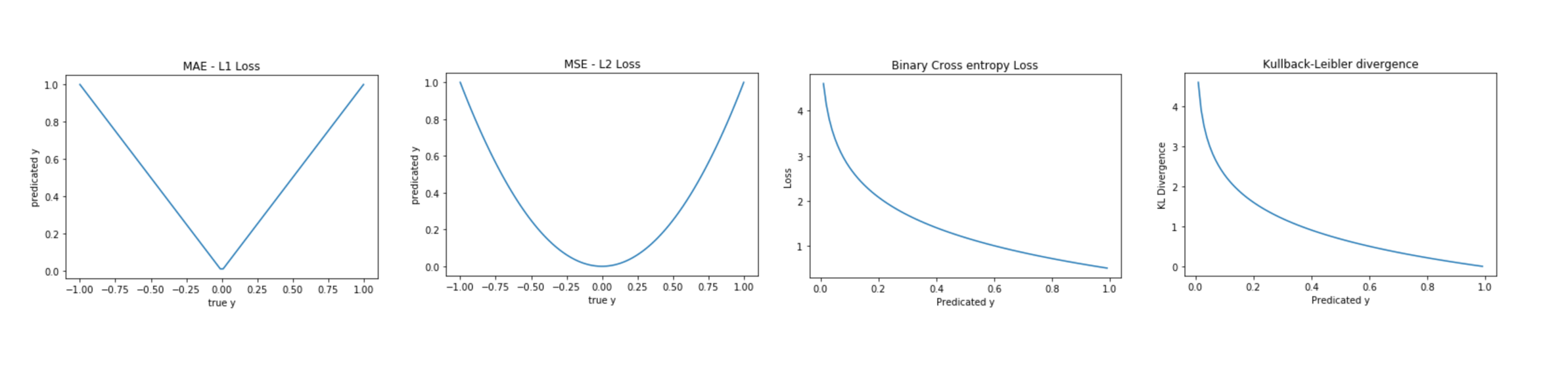Reference

# 3 ways of creating a neural network in PyTorch

## Goal¶

This post aims to introduce 3 ways of how to create a neural network using PyTorch:

Three ways:

• nn.Module
• nn.Sequential
• nn.ModuleList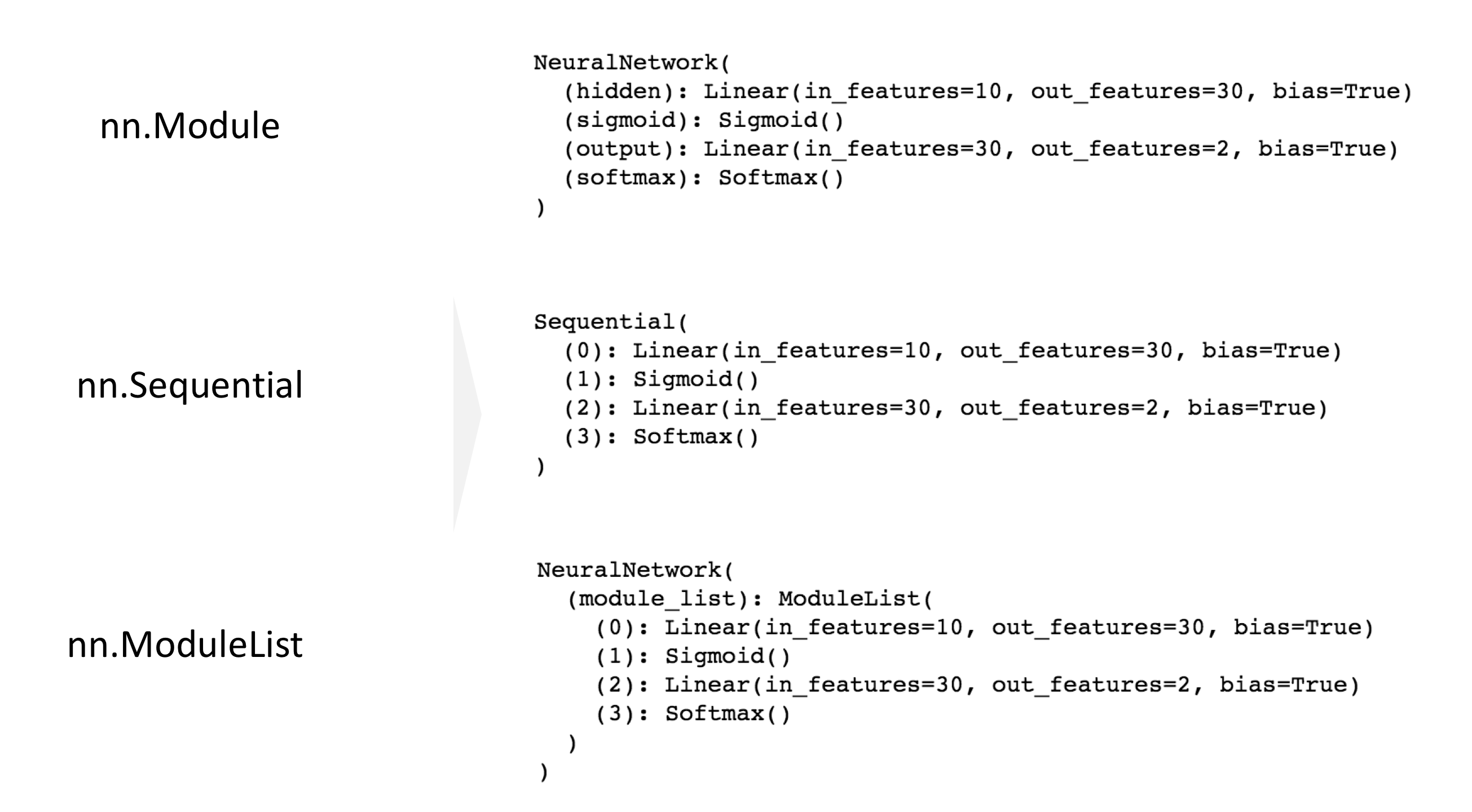Reference

# How to Develop a 1D Generative Adversarial Network From Scratch in PyTorch (Part 1)

## Goal¶

This post is inspired by the blog "Machine Learning Mastery - How to Develop a 1D Generative Adversarial Network From Scratch in Keras" written by Jason Brownlee, PhD. But to learn step-by-step, I will describe the same concept with PyTorch.

This post will cover the followings:

Part 1:

• Select a One-Dimensional Function
• Define a Discriminator Model

Reference

## Libraries¶

In :
import pandas as pd
import numpy as np
import matplotlib.pyplot as plt
%matplotlib inline

# PyTorch
import torch
from torch import nn
from torch import optim
from torchviz import make_dot


## Create a target 1-D function¶

In :
def f(x):
return x **2

In :
n = 100
sigma = 10
x = sigma * (np.random.random(size=n) - 0.5)
plt.plot(x, f(x), '.');
plt.title('Target function $f(x)$');
plt.xlabel('randomly sampled x');
plt.ylabel('$f(x)$');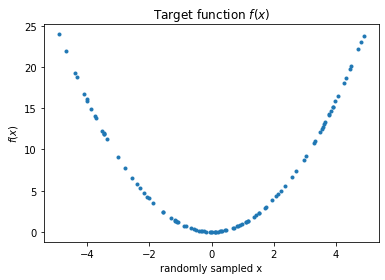## Define a Discriminator Model¶

The definition of a discriminator model is that it will classify the input data into real or fake

In :
# Build a feed-forward network
model = nn.Sequential(nn.Linear(2, 25),
nn.ReLU(),
nn.Sigmoid()
)

# Loss
criterion = nn.CrossEntropyLoss()

# Optimizer

In :
# Visualize this neural network
x = torch.zeros(0, 2, dtype=torch.float, requires_grad=False)
out = model(x)
make_dot(out)

Out:

## Create real and fake samples¶

In :
def generate_samples(size=100, label='real'):
"""Generate samples with real or fake label
"""
x = np.random.randn(size, 1)
x2 = f(x)

y = np.ones((size, 1)) * (label == 'real')
return np.hstack([x, x2]), y


In :
X, y = generate_samples()

In :
x[:5]

Out:
array([[ 1.2483621 ,  1.55840793],
[ 0.57980381,  0.33617245],
[-0.06718955,  0.00451444],
[-1.95352245,  3.81624995],
[-1.14922801,  1.32072501]])
In :
y[:5]

Out:
array([[1.],
[1.],
[1.],
[1.],
[1.]])

# Train the image classifier using PyTorch

## Goal¶

This post aims to introduce how to train the image classifier for MNIST dataset using PyTorch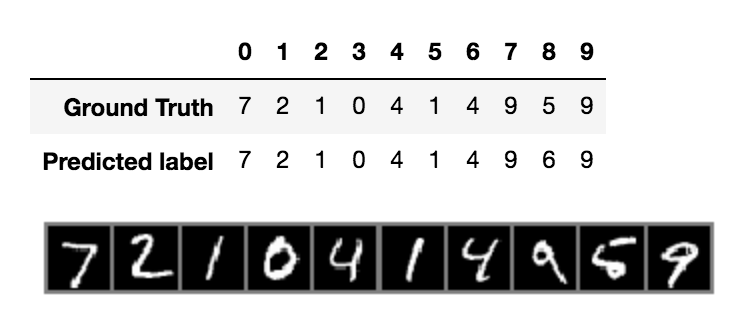Reference

## Libraries¶

In :
import pandas as pd
import numpy as np
import matplotlib.pyplot as plt
%matplotlib inline

# Dataset

# PyTorch
import torch
import torchvision
import torchvision.transforms as transforms

import torch.nn as nn
import torch.nn.functional as F
import torch.optim as optim


## Functions¶

In :
def imshow(img):
img = img / 2 + 0.5 # unnormalize
npimg = img.numpy()
plt.imshow(np.transpose(npimg, (1, 2, 0)))
plt.axis('off')
plt.show()


When downloading the image dataset, we also need to define transform function that apply pixel normalization from [0, 1] to [-1, +1]

In :
transform = transforms.Compose(
[transforms.ToTensor(),
transforms.Normalize((0.5, ), (0.5, ))])

trainset = torchvision.datasets.MNIST(root='~/data',
train=True,
transform=transform)

testset = torchvision.datasets.MNIST(root='~/data',
train=False,
transform=transform)

9920512it [00:28, 1582029.76it/s]

1654784it [00:23, 573182.07it/s]                             

In :
trainloader = torch.utils.data.DataLoader(trainset,
batch_size=100,
shuffle=True,
num_workers=2)

In :
testloader = torch.utils.data.DataLoader(testset,
batch_size=100,
shuffle=False,
num_workers=2)


## Define a model¶

In :
class Net(nn.Module):
def __init__(self):
super(Net, self).__init__()
self.conv1 = nn.Conv2d(1, 32, 3)  # 28x28x32 -> 26x26x32
self.conv2 = nn.Conv2d(32, 64, 3)  # 26x26x64 -> 24x24x64
self.pool = nn.MaxPool2d(2, 2)  # 24x24x64 -> 12x12x64
self.dropout1 = nn.Dropout2d()
self.fc1 = nn.Linear(12 * 12 * 64, 128)
self.dropout2 = nn.Dropout2d()
self.fc2 = nn.Linear(128, 10)

def forward(self, x):
x = F.relu(self.conv1(x))
x = self.pool(F.relu(self.conv2(x)))
x = self.dropout1(x)
x = x.view(-1, 12 * 12 * 64)
x = F.relu(self.fc1(x))
x = self.dropout2(x)
x = self.fc2(x)
return x


## Create a loss function and optimizer¶

In :
model = Net()
criterion = nn.CrossEntropyLoss()
optimizer = optim.SGD(model.parameters(), lr=0.001, momentum=0.9)


## Training a model¶

In :
epochs = 5
for epoch in range(epochs):
running_loss = 0.0
for i, (inputs, labels) in enumerate(trainloader, 0):

# forward + backward + optimize
outputs = model(inputs)
loss = criterion(outputs, labels)
loss.backward()
optimizer.step()

# print statistics
running_loss += loss.item()
if i % 100 == 99:
print(f'[{epoch + 1}, {i+1}] loss: {running_loss / 100:.2}')
running_loss = 0.0

print('Finished Training')

[1, 100] loss: 1.5
[1, 200] loss: 0.82
[1, 300] loss: 0.63
[1, 400] loss: 0.55
[1, 500] loss: 0.5
[1, 600] loss: 0.46
[2, 100] loss: 0.42
[2, 200] loss: 0.4
[2, 300] loss: 0.38
[2, 400] loss: 0.38
[2, 500] loss: 0.34
[2, 600] loss: 0.34
[3, 100] loss: 0.31
[3, 200] loss: 0.31
[3, 300] loss: 0.29
[3, 400] loss: 0.28
[3, 500] loss: 0.28
[3, 600] loss: 0.26
[4, 100] loss: 0.24
[4, 200] loss: 0.24
[4, 300] loss: 0.24
[4, 400] loss: 0.23
[4, 500] loss: 0.23
[4, 600] loss: 0.22
[5, 100] loss: 0.2
[5, 200] loss: 0.21
[5, 300] loss: 0.2
[5, 400] loss: 0.19
[5, 500] loss: 0.19
[5, 600] loss: 0.19
Finished Training


## Test¶

In :
dataiter = iter(testloader)
images, labels = dataiter.next()
outputs = model(images)
_, predicted = torch.max(outputs, 1)

In :
n_test = 10
df_result = pd.DataFrame({
'Ground Truth': labels[:n_test],
'Predicted label': predicted[:n_test]})
display(df_result.T)
imshow(torchvision.utils.make_grid(images[:n_test, :, :, :], nrow=n_test))

0 1 2 3 4 5 6 7 8 9
Ground Truth 7 2 1 0 4 1 4 9 5 9
Predicted label 7 2 1 0 4 1 4 9 6 9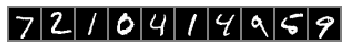# Activation Functions in Neural Networks

## Goal¶

This post aims to introduce activation functions used in neural networks using pytorch.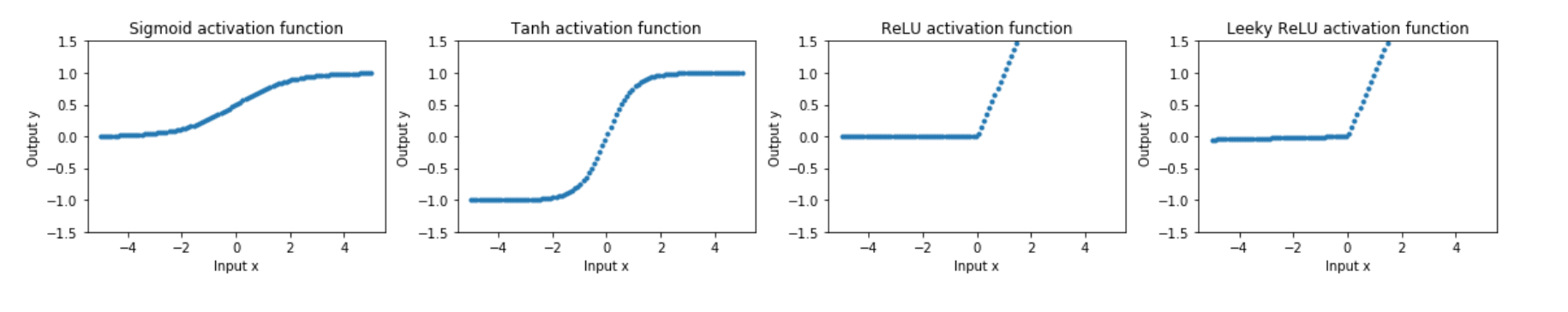Reference

# Style Transfer using Pytorch (Part 4)

## Goal¶

This post aims to explain the concept of style transfer step-by-step. Part 4 is about executing the neural transfer.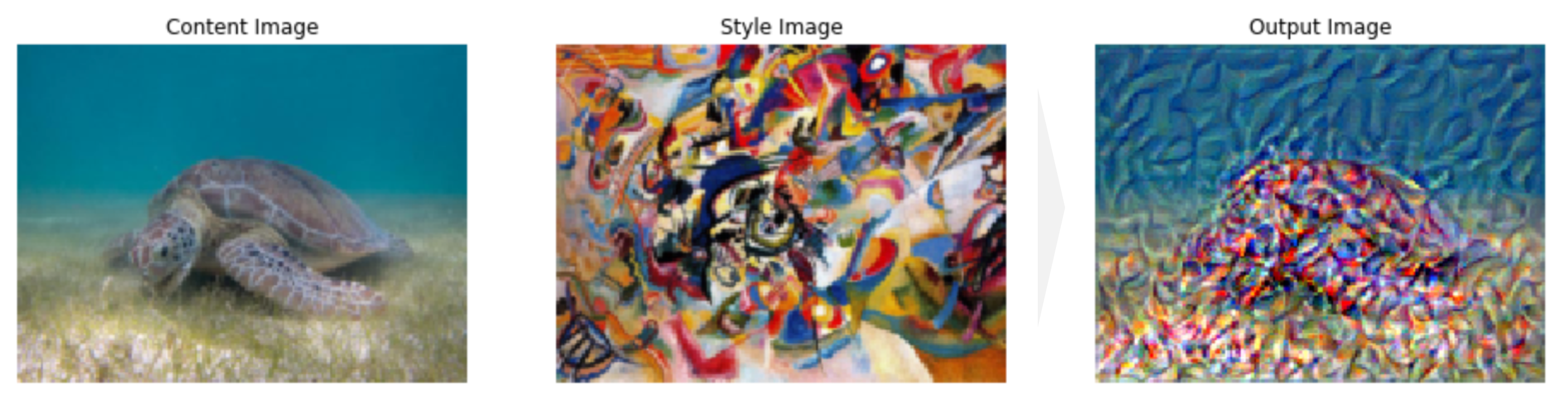Reference

## Libraries¶

In :
import pandas as pd
import copy

# Torch & Tensorflow
import torch
import torch.nn as nn
import torch.nn.functional as F
import torchvision
import tensorflow as tf

# Visualization
from torchviz import make_dot
from PIL import Image
import matplotlib.pyplot as plt
%matplotlib inline

import warnings


## Configuration¶

In :
device = torch.device("cuda" if torch.cuda.is_available() else "cpu")


## Functions¶

The functions covered by the previous posts (Part 1, Part 2, Part 3) are as follows.

### Functions from Part 1 - image loader¶

In :
# desired size of the output image
imsize = (512, 512) if torch.cuda.is_available() else (128, 128)  # use small size if no gpu

torchvision.transforms.Resize(imsize),  # scale imported image
torchvision.transforms.ToTensor()])  # transform it into a torch tensor

image = Image.open(image_name)

# fake batch dimension required to fit network's input dimensions
return image.to(device, torch.float)

In :
unloader = torchvision.transforms.ToPILImage()

def imshow_tensor(tensor, ax=None):
image = tensor.cpu().clone()  # we clone the tensor to not do changes on it
image = image.squeeze(0)      # remove the fake batch dimension

if ax:
ax.imshow(image)
else:
plt.imshow(image)


### Functions from Part 2 - loss functions¶

In :
class ContentLoss(nn.Module):

def __init__(self, target,):
super(ContentLoss, self).__init__()
self.target = target.detach()

def forward(self, input):
self.loss = F.mse_loss(input, self.target)
return input

def gram_matrix(input):
# Get the size of tensor
# a: batch size
# b: number of feature maps
# c, d: the dimension of a feature map
a, b, c, d = input.size()

# Reshape the feature
features = input.view(a * b, c * d)

# Multiplication
G = torch.mm(features, features.t())

# Normalize
G_norm = G.div(a * b * c * d)
return G_norm

class StyleLoss(nn.Module):

def __init__(self, target_feature):
super(StyleLoss, self).__init__()
self.target = gram_matrix(target_feature).detach()

def forward(self, input):
G = gram_matrix(input)
self.loss = F.mse_loss(G, self.target)
return input


### Functions from Part 3 - modeling¶

#### Normalization¶

In :
cnn_normalization_mean = torch.tensor([0.485, 0.456, 0.406]).to(device)
cnn_normalization_std = torch.tensor([0.229, 0.224, 0.225]).to(device)

# create a module to normalize input image so we can easily put it in a
# nn.Sequential
class Normalization(nn.Module):
def __init__(self, mean, std):
super(Normalization, self).__init__()
# .view the mean and std to make them [C x 1 x 1] so that they can
# directly work with image Tensor of shape [B x C x H x W].
# B is batch size. C is number of channels. H is height and W is width.
self.mean = torch.tensor(mean).view(-1, 1, 1)
self.std = torch.tensor(std).view(-1, 1, 1)

def forward(self, img):
# normalize img
return (img - self.mean) / self.std


#### Create a sequential model for style transfer¶

In :
# desired depth layers to compute style/content losses :
content_layers_default = ['conv_4']
style_layers_default = ['conv_1', 'conv_2', 'conv_3', 'conv_4', 'conv_5']

def get_style_model_and_losses(cnn, normalization_mean, normalization_std,
style_img, content_img,
content_layers=content_layers_default,
style_layers=style_layers_default):
cnn = copy.deepcopy(cnn)

# normalization module
normalization = Normalization(normalization_mean, normalization_std).to(device)

# just in order to have an iterable access to or list of content/syle
# losses
content_losses = []
style_losses = []

# assuming that cnn is a nn.Sequential, so we make a new nn.Sequential
# to put in modules that are supposed to be activated sequentially
model = nn.Sequential(normalization)

i = 0  # increment every time we see a conv
for n_child, layer in enumerate(cnn.children()):
#         print()
#         print(f"n_child: {n_child}")
if isinstance(layer, nn.Conv2d):
i += 1
name = 'conv_{}'.format(i)
elif isinstance(layer, nn.ReLU):
name = 'relu_{}'.format(i)
# The in-place version doesn't play very nicely with the ContentLoss
# and StyleLoss we insert below. So we replace with out-of-place
# ones here.
layer = nn.ReLU(inplace=False)
elif isinstance(layer, nn.MaxPool2d):
name = 'pool_{}'.format(i)
elif isinstance(layer, nn.BatchNorm2d):
name = 'bn_{}'.format(i)
else:
raise RuntimeError('Unrecognized layer: {}'.format(layer.__class__.__name__))

#         print(f'Name: {name}')
if name in content_layers:
target = model(content_img).detach()
content_loss = ContentLoss(target)
content_losses.append(content_loss)

if name in style_layers:
target_feature = model(style_img).detach()
style_loss = StyleLoss(target_feature)
style_losses.append(style_loss)

# now we trim off the layers after the last content and style losses
for i in range(len(model) - 1, -1, -1):
if isinstance(model[i], ContentLoss) or isinstance(model[i], StyleLoss):
break

model = model[:(i + 1)]

return model, style_losses, content_losses


In :
d_path = {}

In :
style_img = image_loader(d_path['style'])[:, :, :, :170]
content_img = image_loader(d_path['content'])[:, :, :, :170]
input_img = content_img.clone()

assert style_img.size() == content_img.size(), \
"we need to import style and content images of the same size"


## Modeling¶

In :
# Obtain the model for style transfer
# with warnings.catch_warnings():
warnings.filterwarnings("ignore")
cnn = torchvision.models.vgg19(pretrained=True).features.to(device).eval()
model, style_losses, content_losses = get_style_model_and_losses(cnn, cnn_normalization_mean, cnn_normalization_std, style_img, content_img)


## Executing a neural transfer¶

L-BFGS stands for Limited-memory Broyden–Fletcher–Goldfarb–Shanno according to wiki - Limited-memory_BFGS, which is one of the optimization algorithm using limited amount of memory.

In :
def get_input_optimizer(input_img):
# this line to show that input is a parameter that requires a gradient
return optimizer

optimizer = get_input_optimizer(input_img)


### Execution¶

The execution steps in the function get_style_model_and_losses in NEURAL TRANSFER USING PYTORCH are as follows:

1. Initialization
2. Parameter
3. Define closure function to re-evaluate the model to execute the followings:
• masking images between 0 and 1 by .clamp method
• reset gradient by zero_grad method
• reset the error score for style and content
• compute the style and content loss in each inserted layer
• compute the sum of the losses for style and content
• multiply the weight for style and content to manipulate the style transfer balance by input argument
• execute error back propagation
4. Execute the steps by gradient descent optimizer
In :
# Parameters
num_steps = 10
style_weight=5000
content_weight=1
input_img = content_img[:, :, :, :170].clone()
d_images = {}

print('Building the style transfer model..')
model, style_losses, content_losses = get_style_model_and_losses(cnn,
cnn_normalization_mean, cnn_normalization_std, style_img, content_img)
optimizer = get_input_optimizer(input_img)

# Execution
run = 
while run <= num_steps:

def closure():
# correct the values of updated input image
input_img.data.clamp_(0, 1)

model(input_img)
style_score = 0
content_score = 0

for sl in style_losses:
style_score += sl.loss
for cl in content_losses:
content_score += cl.loss

style_score *= style_weight
content_score *= content_weight

loss = style_score + content_score
loss.backward()

run += 1
if run % 2 == 0:
print("run {}:".format(run))
print('Style Loss : {:4f} Content Loss: {:4f}'.format(
style_score.item(), content_score.item()))
input_img.data.clamp_(0, 1)
d_images[run] = input_img
print()

return style_score + content_score

optimizer.step(closure)

# a last correction...
input_img.data.clamp_(0, 1)

Building the style transfer model..
run :
Style Loss : 1004.939392 Content Loss: 0.000014

run :
Style Loss : 644.263489 Content Loss: 24.647982

run :
Style Loss : 558.792542 Content Loss: 55.995193

run :
Style Loss : 241.166168 Content Loss: 41.970711

run :
Style Loss : 143.137131 Content Loss: 51.402943

run :
Style Loss : 88.965408 Content Loss: 55.758999

run :
Style Loss : 57.654659 Content Loss: 60.926662

run :
Style Loss : 48.282879 Content Loss: 57.995407

run :
Style Loss : 36.090813 Content Loss: 58.100056

run :
Style Loss : 26.983953 Content Loss: 56.346275


In :
fig, axes = plt.subplots(1, 3, figsize=(16, 8))
d_img = {"Content": content_img,
"Style": style_img,
"Output": input_img}
for i, key in enumerate(d_img.keys()):
imshow_tensor(d_img[key], ax=axes[i])
axes[i].set_title(f"{key} Image")
axes[i].axis('off')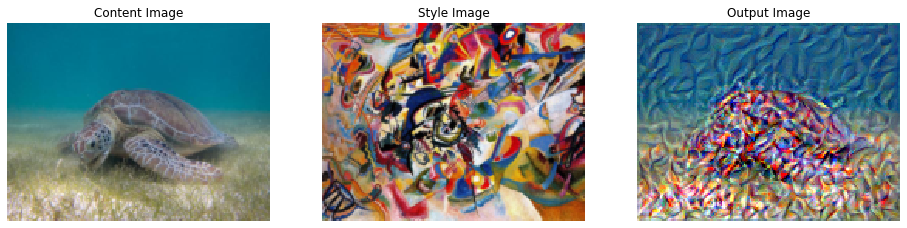### Visualize the process of style transfer¶

This is not yet obvious to see the processes of style transfer. It seems run 2 already finish most of the transfer processes. This needs to be investigated later.

In :
fig, axes = plt.subplots(int(len(d_images)/2), 2, figsize=(16, 20))
for i, key in enumerate(d_images.keys()):
imshow_tensor(d_images[key], ax=axes[i//2][i%2])
axes[i//2][i%2].set_title("run {}:".format(key))
axes[i//2][i%2].axis('off')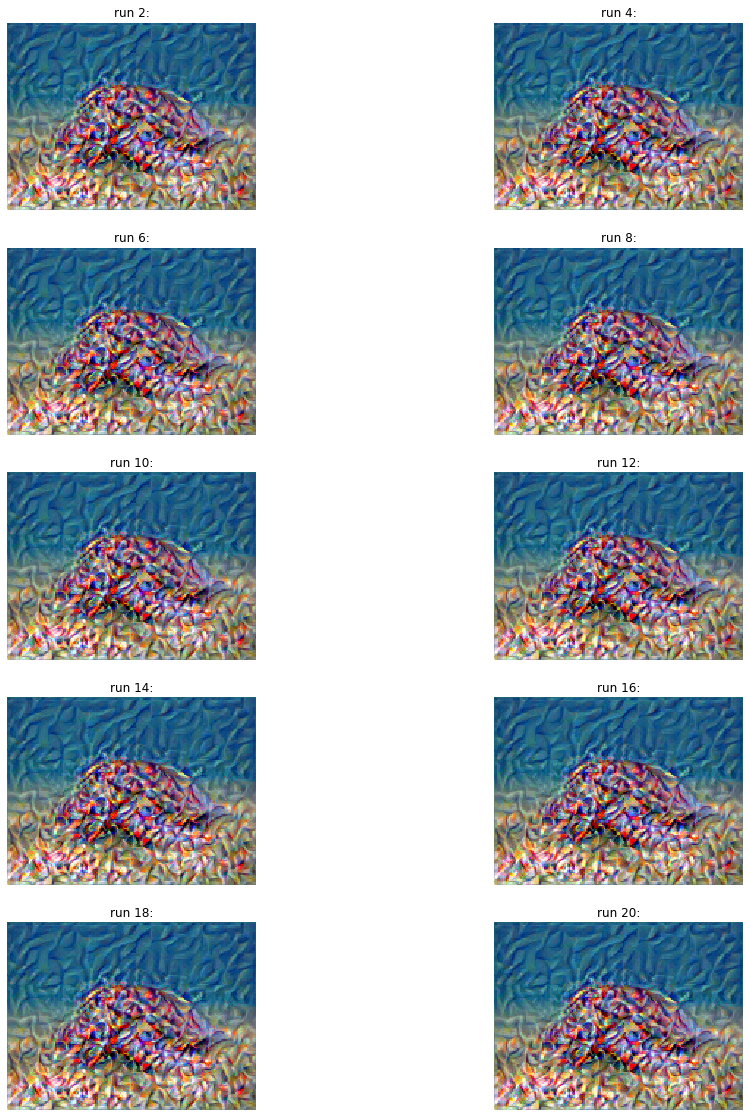# Style Transfer using Pytorch (Part 3)

## Goal¶

This post aims to explain the concept of style transfer step-by-step. Part 3 is about building a modeling for style transfer from VGG19.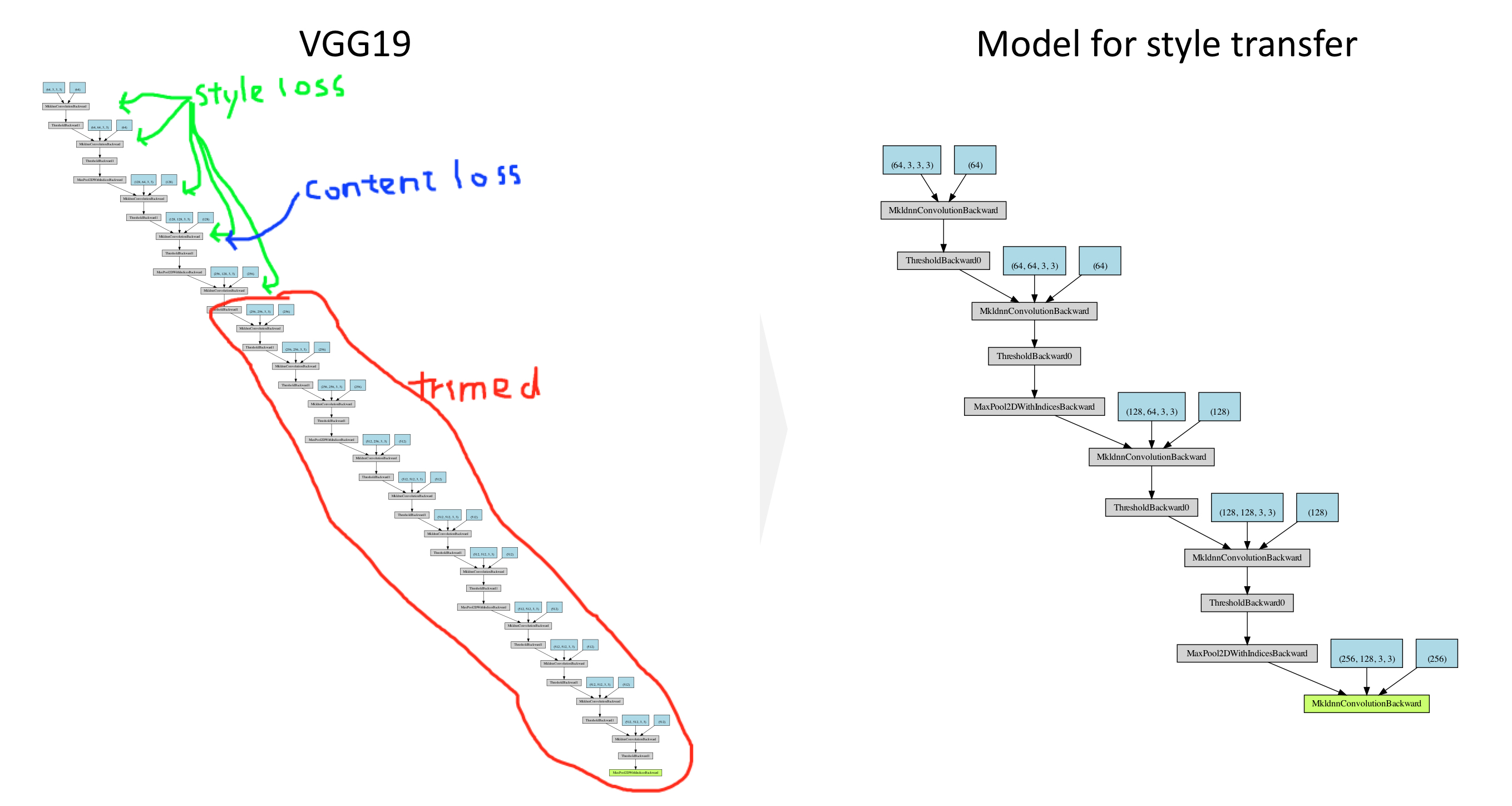Reference

# Style Transfer using Pytorch (Part 2)

## Goal¶

This post aims to explain the concept of style transfer step-by-step. Part 2 is about loss functions.

Reference

## Libraries¶

In :
import pandas as pd

# Torch & Tensorflow
import torch
import torch.nn as nn
import torch.nn.functional as F
import tensorflow as tf

# Visualization
from PIL import Image
import matplotlib.pyplot as plt
%matplotlib inline


## Loss Functions¶

### Content loss¶

Content loss is calculated using MSE (Mean Square Error) between the content images and the output image:

$$MSE = \frac{1}{n} \sum^{n}_{i=1} (Y_{content} - Y_{output})^2$$

# Style Transfer using Pytorch (Part 1)

## Goal¶

This post aims to follow the tutorial NEURAL TRANSFER USING PYTORCH step-by-step. Part 1 is about image loading. The following images for content and style are loaded as PyTorch tensor.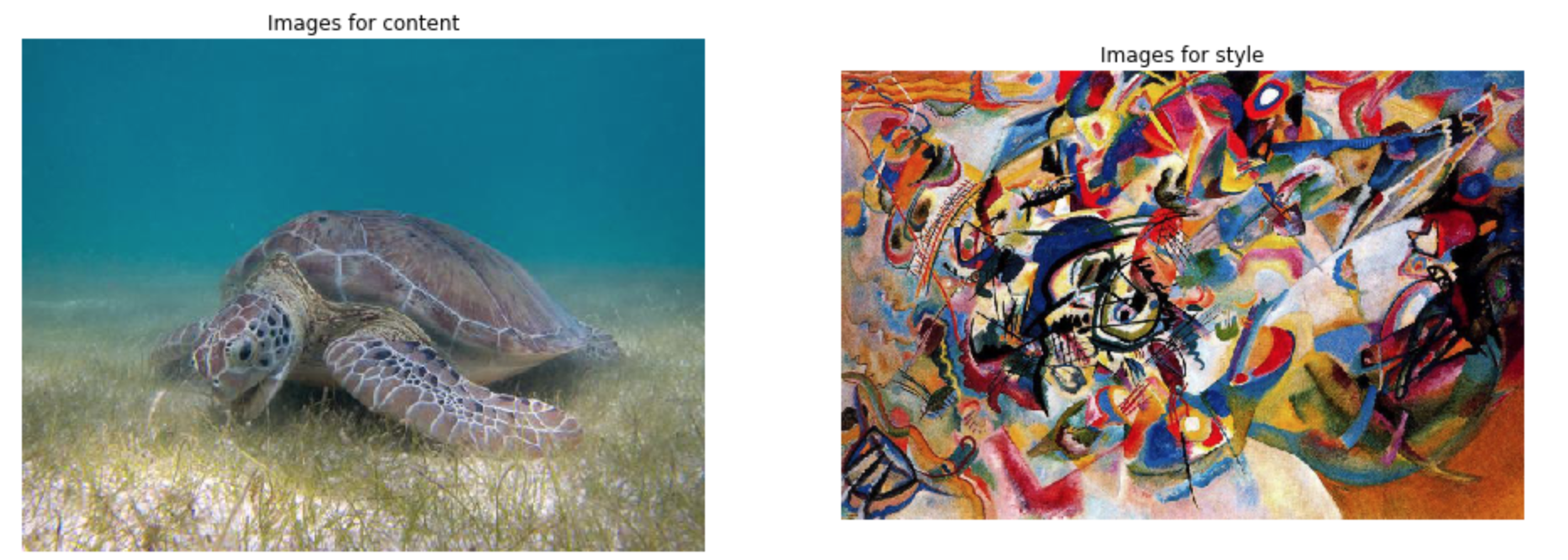Reference